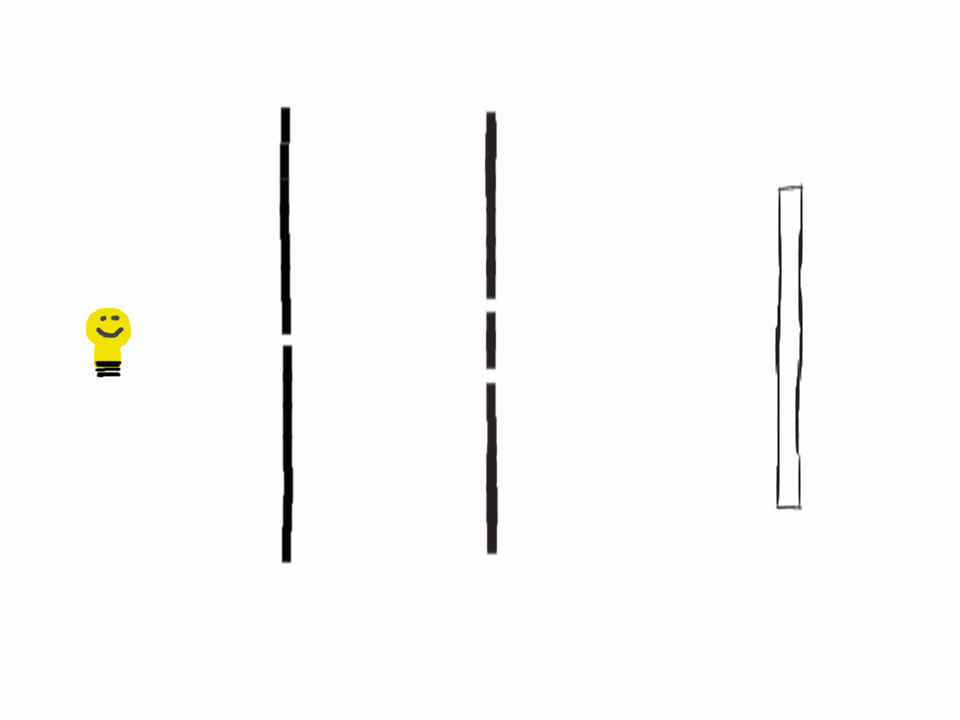#### Introduction to X-Ray DiffractionRemember the double-slit experiment? (Also, remember Microsoft Paint?!) Thomas Young used this experiment to demonstrate the wave-particle duality of light. The slits on the double-slit wall act as point sources, and the light emanating from those sources constructively (and destructively) interfere. In this illustration, the points where the wave fronts intersect are where they constructively interfere.Constructive interference is when the peaks of two waves line up, or intersect, and they add up to an even bigger peak. Destructive interference is when the peak of one wave and the trough of another wave line up or intersect, and they cancel each other out.X-ray diffraction uses the exact same principles of constructive and destructive interference, like in the double-slit experiment. Except now, instead of slits, we use atoms, and instead of visible light, we use x-rays.But before diffraction, there must be scattering. If an x-ray pulse is sufficiently high-energy, then the atom will scatter it through the material. This scattering may occur in any direction with equal probability.Because the scattering direction is random, we like to use lots and lots of x-rays at once so that the scattering occurs in all directions. This is why we use a synchrotron x-ray source instead of the x-ray sources you'd find at a doctor's office.There are different ways we can depict this radially-symmetric distribution of scattered x-rays. In the simplest case, all the x-rays are in phase, and we get a radially-symmetric distribution of alternating amplitude. Let's draw it like this.We can simplify this even further by only concentrating on the peaks. Because we're assuming that the x-rays are all in phase, those points coincide in a circle (or a sphere if we were thinking in three dimensions).Now let's put our atoms together in a "real" material. Here's our unit cell in the corner, which we can assume repeats in all directions many, many times. Then, we aim our x-rays at the material, and we look at the atoms in one plane direction.In diffraction, we're interested in the directions where the x-rays constructively interfere. In this illustration, we can assume that those directions occur toward the points where the scattering circles intersect.But wait, there's two locations where the circles intersect! For each plane type, there are two directions where the x-rays constructively interfere. (Note: the directions on the bottom of the plane would be for the (0 -1 0) plane.)It might look like those directions wouldn't coincide as they are displaced from each other equal to the atom spacing. However, when we zoom way (way, way) out, they form a very focused beam. This is x-ray diffraction!Then, we place a detector behind the sample which is sensitive to x-ray light. In this picture, we see the two spots that resulted from the (010) plane. This is our x-ray diffraction data!